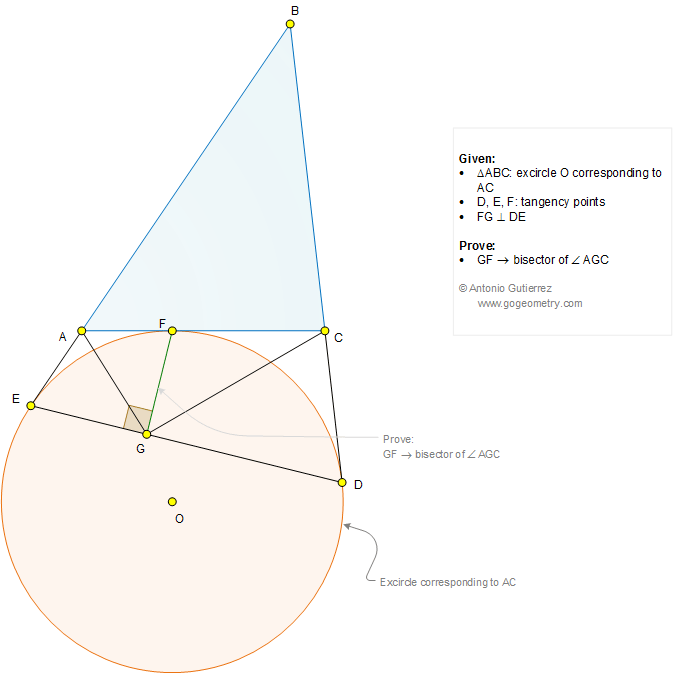# Geometry Problem 1266

### Elements: Triangle, Excircle, Circle, Tangency Points, Perpendicular, 90 Degrees, Angle Bisector

Given a triangle ABC. Let D, E, and F be the points of tangency of the excircle corresponding to AC, as shown in the figure. If FG is perpendicular to DE (G on DE), prove that GF is the bisector of angle AGC.